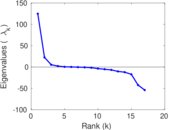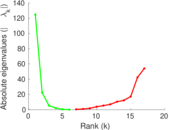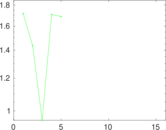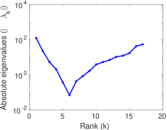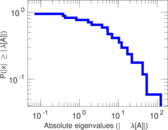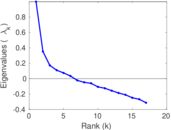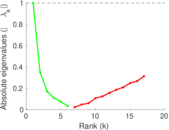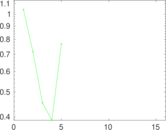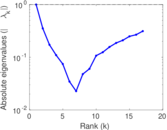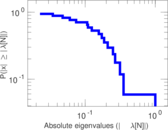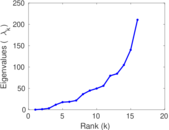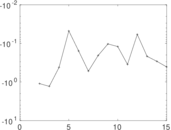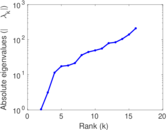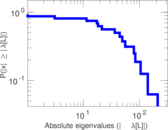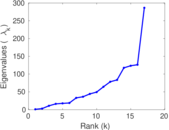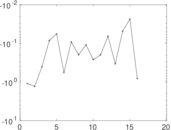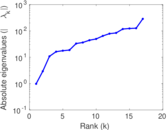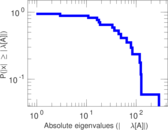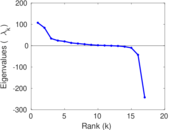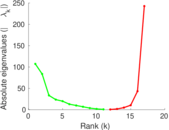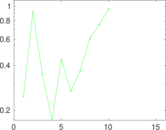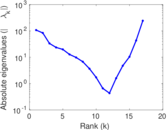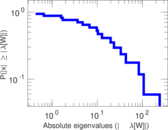# Kangaroos

This undirected network contains interactions between free-ranging eastern grey kangaroos (Macropus giganteus) in the Nadgee Nature Reserve in New South Wales, Australia. A node represents a kangaroo and an edge between two kangaroos shows that there was an interaction between them. The edge weights denote the number of interactions.

 Code `MK` Internal name `moreno_kangaroo` Name Kangaroos Data source http://moreno.ss.uci.edu/data.html#kangaroo AvailabilityDataset is available for download Consistency checkDataset passed all tests Category Animal network Dataset timestamp 1973 Node meaning Kangaroo Edge meaning Interaction Network format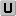Unipartite, undirected Edge type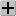Positive weights, no multiple edges LoopsDoes not contain loops

## Statistics

 Size n = 17 Volume m = 91 Loop count l = 0 Wedge count s = 1,010 Claw count z = 3,567 Cross count x = 8,845 Triangle count t = 283 Square count q = 2,114 4-Tour count T4 = 21,134 Maximum degree dmax = 15 Average degree d = 10.705 9 Fill p = 0.669 118 Size of LCC N = 17 Diameter δ = 3 50-Percentile effective diameter δ0.5 = 0.659 794 90-Percentile effective diameter δ0.9 = 1.666 67 Median distance δM = 1 Mean distance δm = 1.227 59 Gini coefficient G = 0.184 228 Balanced inequality ratio P = 0.406 593 Relative edge distribution entropy Her = 0.968 337 Power law exponent γ = 1.451 47 Tail power law exponent γt = 6.071 00 Tail power law exponent with p γ3 = 6.071 00 p-value p = 0.056 000 0 Degree assortativity ρ = −0.193 351 Degree assortativity p-value pρ = 0.008 917 49 Clustering coefficient c = 0.840 594 Spectral norm α = 124.790 Algebraic connectivity a = 1.039 00 Spectral separation |λ1[A] / λ2[A]| = 2.307 23 Non-bipartivity bA = 0.765 565 Normalized non-bipartivity bN = 0.654 378 Algebraic non-bipartivity χ = 0.904 799 Spectral bipartite frustration bK = 0.021 128 5 Controllability C = 0 Relative controllability Cr = 0.000 00

## Plots

### Fruchterman–Reingold graph drawing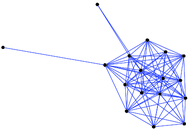### Degree distribution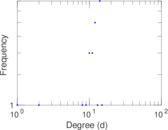### Cumulative degree distribution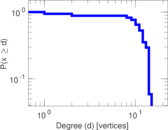### Lorenz curve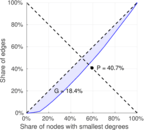### Spectral distribution of the adjacency matrix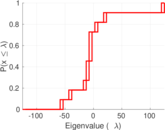### Spectral distribution of the normalized adjacency matrix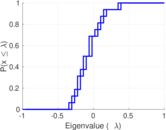### Spectral distribution of the Laplacian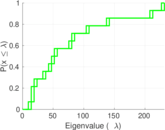### Spectral graph drawing based on the adjacency matrix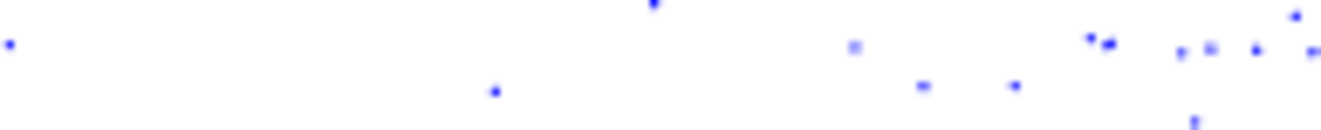### Spectral graph drawing based on the Laplacian### Spectral graph drawing based on the normalized adjacency matrix### Degree assortativity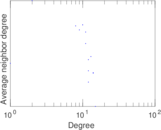### Zipf plot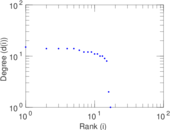### Hop distribution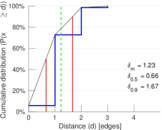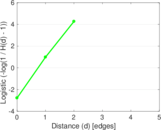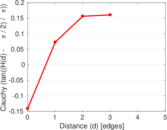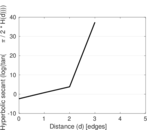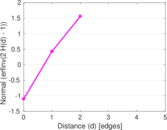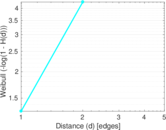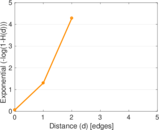### Double Laplacian graph drawing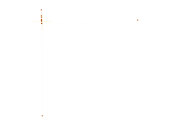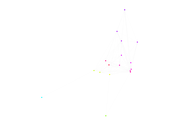### Delaunay graph drawing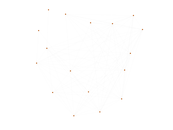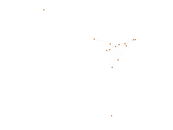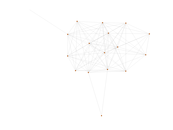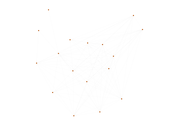### Edge weight/multiplicity distribution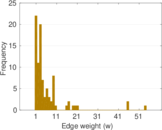### Clustering coefficient distribution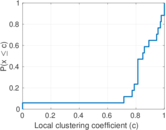### Average neighbor degree distribution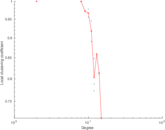### SynGraphy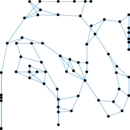### Matrix decompositions plots